New Features in Maple 17: PhysicsSubstantial improvements to the Physics package further enhance Maple’s state-of-the-art environment for algebraic computations in physics.

• New commands for working with tensors and special and general relativity
• A programming library that gives you access to almost 100 internal commands you can use to write your own programs or extend the capabilities of the Physics package
• Enhanced support for vector analysis, Dirac matrices, commutator and anticommutator algebras, and more
• Improved entry and display to make the computational experience even more natural

Details

Tensors and relativity

• A set of five new commands for working in Relativity using tensor notation have been added: ExteriorDerivative, Geodesics, KillingVectors, LieDerivative, and LieBracket. From a conceptual point of view, these commands are all interrelated. This set fills a gap in Maple 16 for working in general relativity using the natural algebraic tensor notation used in Physics without having to perform a reformulation using differential forms.
• Two new commands, Antisymmetrize and Symmetrize, receive a tensorial expression and respectively return the totally antisymmetric and totally symmetric versions of it, obtained performing an average over all permutations of its free indices—in the case of Antisymmetrize with a sign-factor of +1 or -1 according to whether the permutation is even or odd. Specifically, when the expression passed to Antisymmetrize is a product of antisymmetric tensors, the output is also the tensorial version of their exterior product.
• With Physics you can compute with tensors algebraically, with covariant and contravariant indices, as done with paper and pencil, but also with Maple automatically taking into account their symmetry properties, Checking tensor indices in expressions, differentiating and simplifying them using Einstein's sum rule for repeated indices, and automatically setting as contravariant one index in each pair of contracted ones. In Maple 17 the simplification of repeated indices in products has been enhanced in several ways, by identifying symmetric or antisymmetric subexpressions in contracted products and introducing automatic normalization regarding the symmetry properties of user-defined tensors.
• In Maple 17, when you define a tensor —say, you can now define it as an arbitrary tensorial expression involving other tensors, for example simply passingto Define, and the symmetry properties of the tensorial expression will be automatically used to normalize occurrences of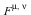in algebraic expressions and when simplifying contracted indices. Moreover, all the internal routines of Physics will handle this new tensor in equal footing with the tensors pre-defined in Physics, including the evaluation when its covariant or contravariant indices assume numerical values and the options for displaying its matrix form or its nonzero components.
• By passing a portion of a metric keyword directly to the metric g_it is possible to list all the database's metrics matches in order to refine a search and choose the desired one. In Maple 17 the database of metrics was enlarged significantly.
• The Riemann tensor command can now return the Riemann invariants for the spacetime metric set - just index Riemann with the keyword invariants instead of with tensor indices, as in Riemann[invariants].
• To represent spacetime coordinates it is possible to use any desired list of names, including predefined cartesian, cylindrical, and spherical lists as in the Physics:-Vectors subpackage, with t representing the time.  Additionally,you can work with many systems of coordinates at the same time. In Maple 17, the SpaceTimeVector formed with these coordinates can also have functional dependency—for example all the coordinates can be functions of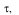the proper time. This is particularly useful when representing or computing Geodesics or working with the 3-D spacelike subset of coordinates.
• It is now possible to save all the Physics settings, set using Setup , during a session and reload them at any timetime. This feature is also available directly from the Setup dialog, which you can launch by calling Setup with no arguments.
• The new Library of 96 programming commands for Physics plus 58 specialized Physics types includes a number of types and commands also useful for interactive computations with tensors, for example, IsTensorialAntisymmetric, IsTensorialSymmetric, and TensorComponents to mention but a few.

Dirac matrices, commutators, anticommutators, and algebras

• The Trace command now works in two different modes. By default, it considers as scalars everything but the algebraic representation for Dirac and Pauli matrices as tensors of one index, or objects explicitly of type matrix or Matrix (Array). The old behavior, where everything but constants were considered traceable objects can be restored with the new Setup option traceonlymatrices = false (its default value is true). This permits using Trace more naturally in the typical case, which is the computation of traces of expressions involving Dirac matrices where the coefficients are not just constants.
• When Commutator and AntiCommutator algebras are set using Setup, a basic test for consistency of the algebra rules set is performed and an interruption with instructive messages happens when inconsistency is detected. Likely, some algebra rules automatically imply on the anticommutativity or noncommutativity of the related operands, and so these operands are automatically set as anticommutative or noncommutative accordingly.
• It is now possible to define unitary operators in equal footing with quantum and hermitian operators. When unitary operators are set, Setup automatically creates algebra rules for them.  These rules are automatically taken into account by Simplify when simplifying products involving these operators.
• The conjugate and Dagger of a tensor and a quantum operator, say A, is now handled, taking into account any algebra rules for A as determined by Setup. In FeynmanDiagrams the conjugate spinor can now also be represented using conjugate or Dagger of an anticommutative field. Likewise, the system now knows better how to handle function compositions involving Bracket, conjugate Dagger, Trace, and Inverse of quantum operators.

Vector analysis

• All the vector analysis routines of Physics:-Vectors can compute with unit vectors and geometrical coordinates that have functional dependency, as in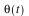or the unit vector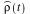, enlarging the mathematical representation capabilities. New in Maple 17, the relationships between cartesian, cylindrical, and spherical coordinates as well as between these coordinates and the related unit vectors in the three systems are automatically taken into account when differentiating. Additionally, propagation of functionality in these geometric relationships, naturally done in our minds when computing with paper and pencil, is now also done by the computer. So in a simple example, if an expression involves, the relationship taken automatically into account when differentiating w.r.t.is notbut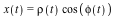.
• In connection with these changes, the Maple 16 command Physic:-Vectors:-VectorDiff was renamed Physic:-Vectors:-diff and is now the same one as Physic:-diff. In turn this single diff command now accepts an optional argument, geometricdifferentiation, to take into account these geometric relationships mentioned between coordinates and unit vectors. This feature of the new single diff is automatically turned ON when Vectors is loaded, but can be turned ON and OFF at any moment using Setup (keyword geometricdifferentiation).

A new library of programming commands for Physics

• A new programming library with 96 commands plus 58 specialized Physics types has been added to Physics, presenting at the user-level a useful selection of the internal routines and types used at Maplesoft to program the Physics commands. You can now use these programming tools to write your own programs or extend the capabilities of the Physics package, without having to program everything from the scratch, and in a way that is entirely compatible with the existing package. In this way your programs can also interact with the ones in Physics directly.
• Many of the programming tools in Physics:-Library are also useful for interactive computation. To mention but a few, AntiCommute and Commute respectively tell whether two objects anticommute or commute; IsTensorialAntisymmetric and IsTensorialSymmetric respectively tell whether a given tensorial expression is totally antisymmetric or totally symmetric with respect to permutations of its free indices, or regarding any particular pair of them; Backup and Restore respectively create a backup of all the settings of Physics at some moment, and restore those settings after performing any exploratory computations that could have changed those settings (for example, redefining quantum operators using algebra rules or changing the dimension of spacetime or its metrics).